CAT  >  Practice Questions Level 2: Exponents & Logarithm - 2

# Practice Questions Level 2: Exponents & Logarithm - 2 - Notes | Study Level-wise Practice Questions for CAT Preparation - CAT

 1 Crore+ students have signed up on EduRev. Have you?

This EduRev document offers 15 Multiple Choice Questions (MCQs) from the topic Exponents & Logarithm (Level - 2). These questions are of Level - 2 difficulty and will assist you in the preparation of CAT & other MBA exams. You can practice/attempt these CAT Multiple Choice Questions (MCQs) and check the explanations for a better understanding of the topic.

Question for Practice Questions Level 2: Exponents & Logarithm - 2
Try yourself:If x + 1/x = 3, then the value of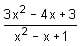is

Question for Practice Questions Level 2: Exponents & Logarithm - 2
Try yourself:If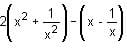– 7 = 0, then two values of x are

Question for Practice Questions Level 2: Exponents & Logarithm - 2
Try yourself:Find the value of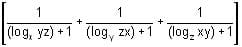.

Question for Practice Questions Level 2: Exponents & Logarithm - 2
Try yourself:Which of the following is true?

Question for Practice Questions Level 2: Exponents & Logarithm - 2
Try yourself:If x = log24 + log35 + log46 + log57 + log68 + log79, then which of the following is true?

Question for Practice Questions Level 2: Exponents & Logarithm - 2
Try yourself:If1/3 log3 M + 3 log3 N = 1 + log0.008 5, then

Question for Practice Questions Level 2: Exponents & Logarithm - 2
Try yourself:If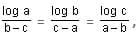then find the value of aa bb cc.

Question for Practice Questions Level 2: Exponents & Logarithm - 2
Try yourself:If log10 x - log10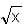= 2 logx 10, then x is equal to

Question for Practice Questions Level 2: Exponents & Logarithm - 2
Try yourself:If log (a + c), log (c - a), log (a - 2b + c) are in A.P, then

Question for Practice Questions Level 2: Exponents & Logarithm - 2
Try yourself:Arrange the following in descending order:

A = 1822, B = 2218, C = 2117

Question for Practice Questions Level 2: Exponents & Logarithm - 2
Try yourself:The sum of the series x2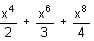+ ..., |x| < 1, is

Question for Practice Questions Level 2: Exponents & Logarithm - 2
Try yourself:Find the value of x in log (x - 2) + log (x - 1) = log 20 - 1.

Question for Practice Questions Level 2: Exponents & Logarithm - 2
Try yourself:If log12 27 = a and log9 16 = b, then find log8 108.

Question for Practice Questions Level 2: Exponents & Logarithm - 2
Try yourself: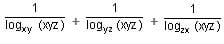equals

Question for Practice Questions Level 2: Exponents & Logarithm - 2
Try yourself:Let u = (log2 x)2 - 6 log2 x + 12, where x is a real number. Then, the equation xu = 256 has

The document Practice Questions Level 2: Exponents & Logarithm - 2 - Notes | Study Level-wise Practice Questions for CAT Preparation - CAT is a part of the CAT Course Level-wise Practice Questions for CAT Preparation.
All you need of CAT at this link: CAT

## Level-wise Practice Questions for CAT Preparation

277 docs
 Use Code STAYHOME200 and get INR 200 additional OFF

## Level-wise Practice Questions for CAT Preparation

277 docs

### How to Prepare for CAT

Read our guide to prepare for CAT which is created by Toppers & the best Teachers

Track your progress, build streaks, highlight & save important lessons and more!

,

,

,

,

,

,

,

,

,

,

,

,

,

,

,

,

,

,

,

,

,

;